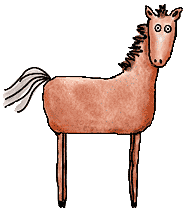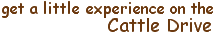# Assignment Java-7 (Sum)

Purpose: The purpose of this particular assignment is to learn the boundaries of double (precision).

Write a program that will start with a double called sum set to zero. Add 0.1 to sum 1000 times by using a for loop.

Print the sum, and then print a statement of whether or not the sum is equal to 100.

To do this, you will need to know:

After you have tied up this assignment, lasso the next one:

### Assignment Java-8 (GeekWatch)

Page maintained by Marilyn de Queiroz